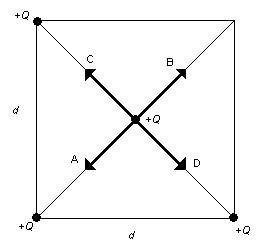# College Physics : Coulomb's Law

## Example Questions

### Example Question #1 : Electrostatics

When the magnitude of two interacting charges is increased by a factor of 2, the electrical forces between these charges is __________.

reduced by a factor ofdoubled

reduced by a factor of 3

reduced by a factor of 4

Explanation:

In Coloumb's law, an increase in both interacting charges will cause an increase in the magnitude of the electrical force between them. Specifically if the magnitude of both interacting charges is doubled, this will quadruple the electrical force.

### Example Question #11 : Electromagnetics, Waves, And Optics

What is the main difference between electrical and gravitational forces?

Gravitational forces are always attractive but electrical forces can be attractive or repulsive

Gravitational forces obey the inverse square law and electrical forces do not

Electrical forces obey the inverse square law and gravitational forces do not

Electrical forces attract and gravitational forces repel

Gravitational forces are always attractive but electrical forces can be attractive or repulsive

Explanation:

Electric forces can be attractive or repulsive because charges may be positive or negative. In the case for gravitational forces, there are only attractive forces because mass is always positive.

### Example Question #1 : ElectrostaticsThree equal charges are at three of the corners of a square of side d. A fourth charge of equal magnitude is at the center of the square as shown in Figure above. Which of the arrows shown represents the net force acting on the charge at the center of the square?

D

A

B

C

B

Explanation:

Because of the principles of superposition, each electric force that acts from the charges at the corners on to the charge at the center can be broken into components. Since all the charges are positive, all the forces will be repulsive. The forces acting from the top left and bottom right corners will cancel, leaving only the repulsive force coming from the bottom left corner.

### Example Question #1 : Coulomb's Law

An electron traveling along the +x-axis enters an electric field that is directed vertically down, i.e., along the negative y-axis. What will be the direction of the electric force acting on the electron after entering the electric field?

Out of the page

Into the page

Upward

To the right

Upward

Explanation:

Positive charges in an electric field will experience an electric force that is in the same direction as the electric field. If the charge is negative, the force will be in the opposite direction of the electric field. Since we are talking about an electron moving in an electric field that points in the negative y-direction, the electron will feel a force that points in the positive y-direction, or upwards.

### Example Question #1 : Coulomb's Law

A charged rod carrying a negative charge is brought near two spheres that are in contact with each other but insulated from the ground. If the two spheres are then separated, what kind of charge will be on the spheres?

The spheres do not get any charge

None of these

The sphere near the charged rod becomes negative and the other becomes positive

The sphere near the charged rod becomes positive and the other becomes negative

The sphere near the charged rod becomes positive and the other becomes negative

Explanation:

When the negatively charged rod is brought near one of the two spheres, the presents of the negative charge will induce a flow of charge in the spheres such that regions farthest away from the charged rod will become most negative and regions near the rod will become most positive. This is called charge by induction.

### Example Question #1 : Electrostatics

By what method will a positively charged rod produce a negative charge on a conducting sphere that is placed on an insulating surface?

Charge by conduction

Charge by induction

None of these

Charge by convection